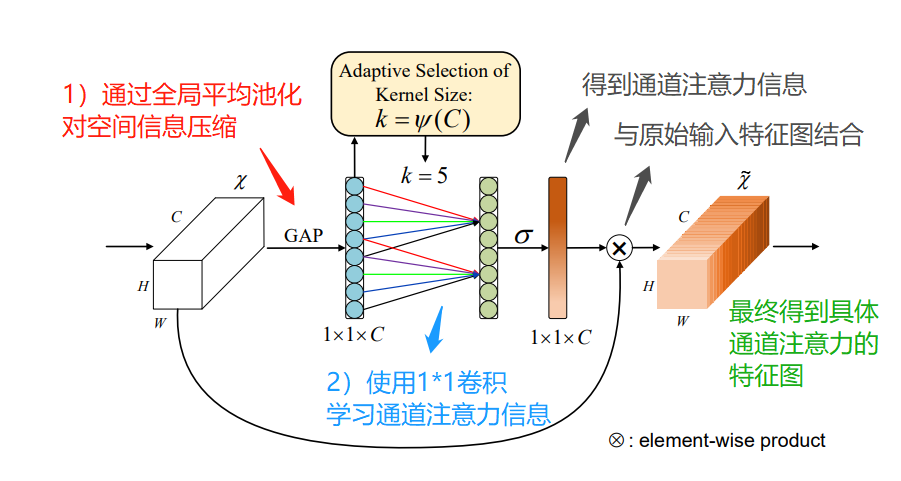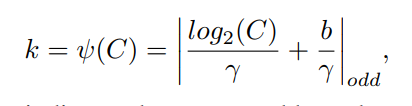12月 6, 2023

Processing Machinery

# ECA Attention Module Principle Analysis and Code Implementation# Foreword

This article introduces the ECA attention module, which was proposed in ECA-Net, which is a paper in 2020 CVPR; the ECA module can be used in the CV model and can extract model accuracy, so I will introduce its principle to you , design ideas, code implementation, and how to apply it in the model.

## 1. ECA attention module

ECA attention module, which is a channel attention module; often used in vision models. It supports plug-and-play, that is, it can enhance the channel features of the input feature map, and the final output of the ECA module does not change the size of the input feature map.

Background: ECA-Net believes that: the dimensionality reduction operation adopted in SENet will have a negative impact on the prediction of channel attention; it is inefficient and unnecessary to obtain the dependencies of all channels at the same time;
Design: Based on the SE module, ECA uses the fully connected layer FC to learn channel attention information in SE, and changes it to 1*1 convolution learning channel attention information;
Function: Use 1*1 convolution to capture information between different channels, avoid channel dimension reduction when learning channel attention information; reduce the amount of parameters; (FC has a large amount of parameters; 1*1 convolution has only a small parameter amount)
Let’s analyze how ECA realizes channel attention; first, let’s look at the structure of the module:The process idea of the ECA model is as follows:

First input the feature map, its dimension is H*W*C;
Perform spatial feature compression on the input feature map; implementation: in the spatial dimension, use the global average pooling GAP to obtain a 1*1*C feature map;
Carry out channel feature learning on the compressed feature map; realize: through 1*1 convolution, learn the importance between different channels, and the output dimension at this time is still 1*1*C;
Finally, the channel attention is combined, and the feature map 1*1*C of channel attention and the original input feature map H*W*C are multiplied channel by channel, and finally the feature map with channel attention is output.

2. 1*1 convolution learning channel attention information

Note, this part is the key! Note, this part is the key! Note, this part is the key!

First of all, recall that when using the FC fully connected layer, the input channel feature map is processed globally for learning;

If 1*1 convolution is used, only the information between local channels can be learned;

Then the question comes, if the input channel feature map has a relatively large size, that is, a 1*1*C feature map, it has a large number of channels; is it appropriate to use a small convolution kernel to perform a 1*1 convolution operation? ?

It is obviously not suitable, and a larger convolution kernel should be used to capture more information between channels.

Similarly, if the input channel feature map is relatively small in size, it is not appropriate to use a large convolution kernel to perform a 1*1 convolution operation.

When performing convolution operations, the size of its convolution kernel will affect the receptive field; in order to solve different input feature maps and extract features of different ranges, ECA uses a dynamic convolution kernel to do 1*1 convolution , to learn the importance among different channels.

The dynamic convolution kernel means that the size of the convolution kernel adapts to changes through a function;

In the layer with a large number of channels, use a larger convolution kernel to perform 1*1 convolution, so that more cross-channel interactions can be performed;
In the layer with a small number of channels, use a smaller convolution kernel to do 1*1 convolution, so that there is less cross-channel interaction;
Convolution and adaptation functions, defined as follows:Where k represents the size of the convolution kernel; C represents the number of channels; | |odd represents that k can only take an odd number; \gamma and b represent that they are set to 2 and 1 in the paper, which are used to change the number of channels C and the size of the convolution kernel and between proportion.

3. Code implementation

ECA channel attention module, the code based on the pytorch version is as follows:

class ECABlock(nn.Module):
def __init__(self, channels, gamma = 2, b = 1):
super(ECABlock, self).__init__()

# 设计自适应卷积核，便于后续做1*1卷积
kernel_size = int(abs((math.log(channels, 2) + b) / gamma))
kernel_size = kernel_size if kernel_size % 2 else kernel_size + 1

# 全局平局池化

# 基于1*1卷积学习通道之间的信息
self.conv = nn.Conv1d(1, 1, kernel_size = kernel_size, padding = (kernel_size – 1) // 2, bias = False)

# 激活函数
self.sigmoid = nn.Sigmoid()

def forward(self, x):
# 首先，空间维度做全局平局池化，[b,c,h,w]==>[b,c,1,1]
v = self.avg_pool(x)

# 然后，基于1*1卷积学习通道之间的信息；其中，使用前面设计的自适应卷积核
v = self.conv(v.squeeze(-1).transpose(-1, -2)).transpose(-1, -2).unsqueeze(-1)

# 最终，经过sigmoid 激活函数处理
v = self.sigmoid(v)
return x * v

4. ECA is applied in the model

The ECA module can be used in the CV model, which can effectively extract the model accuracy; it is plug-and-play, and its usage is similar to that of the SE model.

Application example 1:

In the backbone network (Backbone), ECA modules are added to enhance channel characteristics and improve model performance;

Application example 2:

At the end of the backbone network (Backbone), the ECA model is added to enhance the overall channel characteristics and improve model performance;

Application example 3:

In the multi-scale feature branch, the ECA module is added to strengthen channel features and improve model performance.

Overall evaluation: ECA is very similar to SE. It just changes the FC fully connected layer to 1*1 convolution when learning channel attention information; there are fewer parameters, but the model improvement effect is not necessarily as good as the SE module.